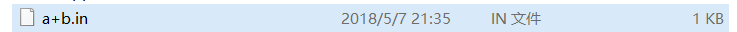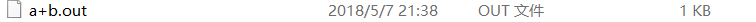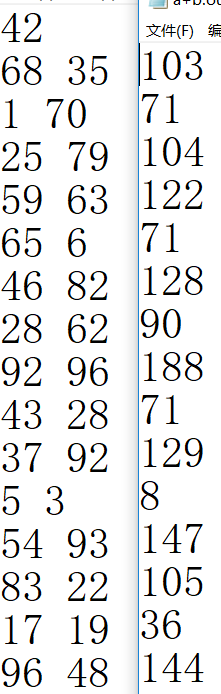## C语言读写文件的操作

```# include <stdio.h>
# include <stdlib.h>

int main(void)
{
int k, n, m;
freopen("a+b.in", "w", stdout);   //将要测试的数据写入到一个文件内,这个文件会在编译的时候生成,就和cpp文件在同一个地方。
k = rand()%100+1;  //随机生成一个数,为测试的数据
printf("%d\n", k);
while (k --)
{
n = rand()%100+1;  // 读入2个整数.
m = rand()%100+1;
printf("%d %d\n", n, m);
}
fclose(stdout);   //结束写入文件.
return 0;
}``````# include <stdio.h>

int main(void)
{
int a, b, c;
freopen("a+b.in", "r", stdin);   // 读入刚写的文件
freopen("a+b.out", "w", stdout);  // 输出将要输出的数据

scanf("%d", &c);
while (c --)
{
scanf("%d %d", &a, &b);
printf("%d\n", a+b);
}

fclose(stdin);   //结束
fclose(stdout);	  //结束
return 0;
}```